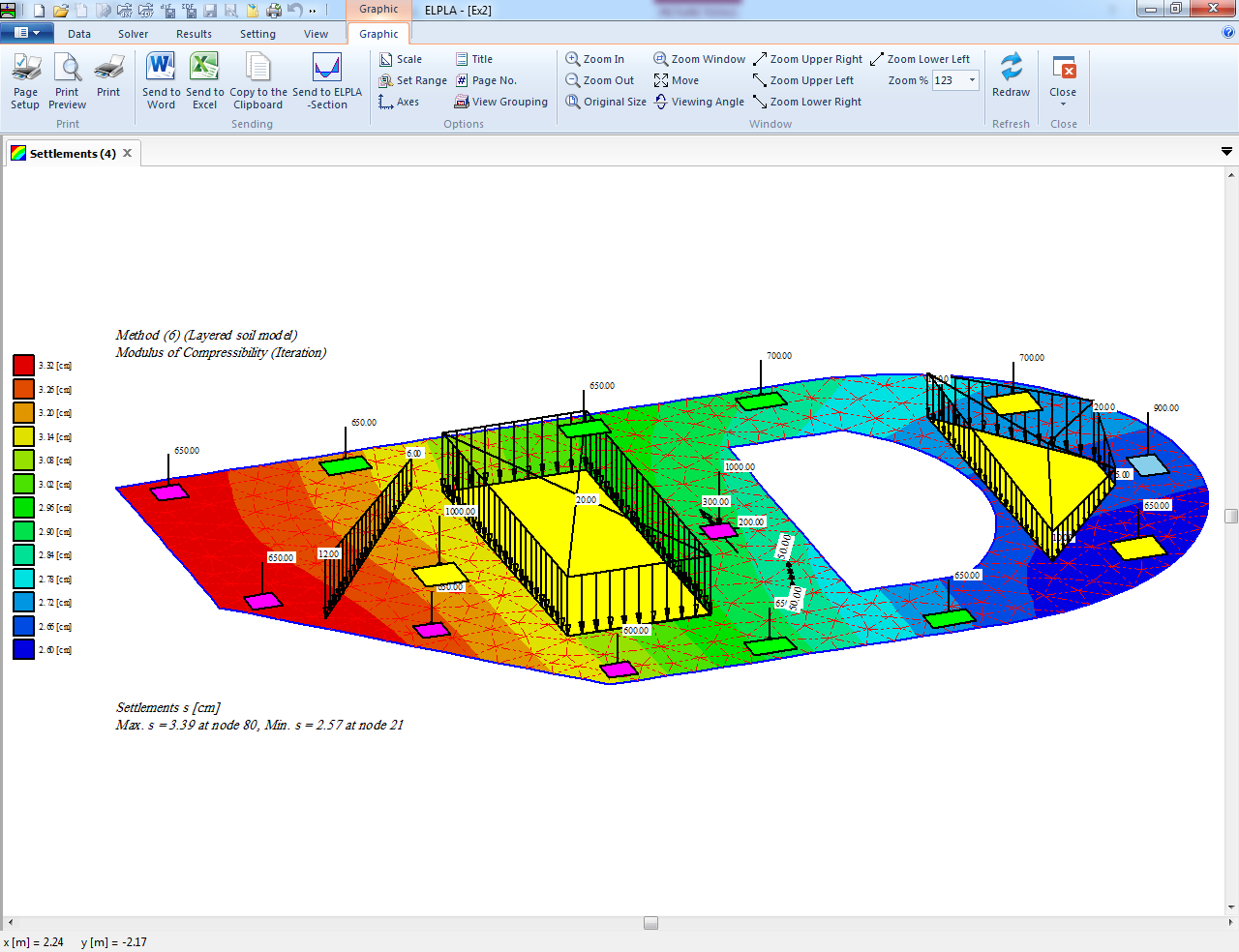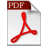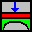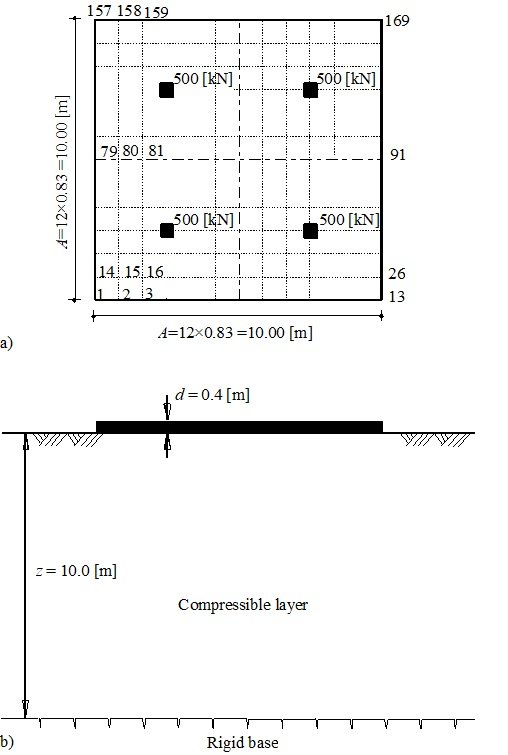Analysis of Shallow Foundations
ELPLA (ELastic PLAte) is a software which is used for analyzing geotechnical and structure problems. One of them is analyzing shallow foundations with the real subsoil model.

• The purpose of this text is to present the methods, equations, procedures, and techniques used in the formulation and development of the ELPLA function. It is of value to be familiar with this information when using the software
• An understanding of these concepts will be of great benefit in applying the software, resolving difficulties and judging the acceptability of the results
• Two familiar types of subsoil models are considered, Winkler’s model and Continuum model. In addition, the simple assumption model is also considered. The model assumes linear contact pressure on the base of the foundation
• Finite elements-method is used to analyze both of the raft and grid foundations (or the ribbed raft). In which plate bending elements represent the raft according to the two-dimensional nature of foundation, while grid elements represent the gridDocuments and DataComputer Analysis of Foundations by ELPLAELPLA-Data Files of Shallow Foundations
2.1 Analysis of a square raft on irregular subsoil

This example is carried out to show the influence of irregular subsoil on the values of settlements, contact pressures and moments.

The analysis of the square raft is carried out by the two familiar types of soil models: Winkler’s and Continuum models for elastic foundations, besides the analysis of rigid raft on Continuum model, using the following three calculation methods:

Method 3         Variable modulus of subgrade reaction method

Method 7         Modulus of compressibility method

Method 8         Rigid raft on compressible subsoil

A square raft of 10 [m] side is subdivided into 144 square elements. The raft thickness is d = 0.4 [m].2.1 Analysis of a square raft on irregular subsoilELPLA-Square raft on irregular subsoil